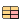## Buildings.HeatTransfer.Data.BaseClasses

Base classes for package Data

### Information

Extends from Modelica.Icons.BasesPackage (Icon for packages containing base classes).

### Package Content

Name DescriptionMaterial Thermal properties of materials w/o storageThermalProperties Thermal properties of materials with storage

##Buildings.HeatTransfer.Data.BaseClasses.Material

Thermal properties of materials w/o storage

### Information

Base record for materials that declares the thermal properties.

The specific heat capacity can be zero, in which case the material will be modeled as a thermal resistor that does not store energy.

Note that the thermal resistance is in units of m2 K ⁄ W and not K ⁄ W because this record does not have the surface area as a parameter. The surface area will be defined in the model of the construction that uses this material. This allows use of the same material in walls, floors and ceilings of different surface area.

Extends from Modelica.Icons.Record (Icon for records).

### Parameters

TypeNameDefaultDescription
Lengthx Material thickness [m]
ThermalConductivityk Thermal conductivity [W/(m.K)]
SpecificHeatCapacityc Specific heat capacity [J/(kg.K)]
Densityd Mass density [kg/m3]
RealR Thermal resistance of a unit area of material [m2.K/W]
IntegernStaRef3Number of state variables in a reference material of 0.2 m concrete
BooleansteadyState(c < Modelica.Constants.eps ...Flag, if true, then material is computed using steady-state heat conduction
Properties for phase change material
TemperatureTSol Solidus temperature, used only for PCM. [K]
TemperatureTLiq Liquidus temperature, used only for PCM [K]
SpecificInternalEnergyLHea Latent heat of phase change [J/kg]
IntegernStamax(1, integer(ceil(nStaReal...Actual number of state variables in material
RealpiRef331.4Ratio x/sqrt(alpha) for reference material of 0.2 m concrete
RealpiMatif steadyState then piRef el...Ratio x/sqrt(alpha)
RealnStaRealnStaRef*piMat/piRefNumber of states as a real number

### Modelica definition

record Material "Thermal properties of materials w/o storage" extends Modelica.Icons.Record; parameter Modelica.Units.SI.Length x "Material thickness"; parameter Modelica.Units.SI.ThermalConductivity k "Thermal conductivity"; parameter Modelica.Units.SI.SpecificHeatCapacity c "Specific heat capacity"; parameter Modelica.Units.SI.Density d "Mass density"; parameter Real R(unit="m2.K/W") "Thermal resistance of a unit area of material"; parameter Integer nStaRef(min=0) = 3 "Number of state variables in a reference material of 0.2 m concrete"; parameter Integer nSta(min=1)=max(1, integer(ceil(nStaReal))) "Actual number of state variables in material"; parameter Boolean steadyState= (c < Modelica.Constants.eps or d < Modelica.Constants.eps) "Flag, if true, then material is computed using steady-state heat conduction"; parameter Real piRef=331.4 "Ratio x/sqrt(alpha) for reference material of 0.2 m concrete"; parameter Real piMat=if steadyState then piRef else x*sqrt(c*d/k) "Ratio x/sqrt(alpha)"; parameter Real nStaReal(min=0) = nStaRef*piMat/piRef "Number of states as a real number"; parameter Modelica.Units.SI.Temperature TSol "Solidus temperature, used only for PCM."; parameter Modelica.Units.SI.Temperature TLiq "Liquidus temperature, used only for PCM"; parameter Modelica.Units.SI.SpecificInternalEnergy LHea "Latent heat of phase change"; constant Boolean ensureMonotonicity = false "Set to true to force derivatives dT/du to be monotone"; constant Boolean phasechange = false "Flag, true if the material is a phase change material"; end Material;

##Buildings.HeatTransfer.Data.BaseClasses.ThermalProperties

Thermal properties of materials with storage

### Information

Base record for materials, used in circular geometry or other configurations, that only declares the thermal properties.

The specific heat capacity can be zero, in which case the material will be modeled as a thermal resistor that does not store energy.

Extends from Modelica.Icons.Record (Icon for records).

### Parameters

TypeNameDefaultDescription
ThermalConductivityk Thermal conductivity [W/(m.K)]
SpecificHeatCapacityc Specific heat capacity [J/(kg.K)]
Densityd Mass density [kg/m3]
BooleansteadyState(c < Modelica.Constants.eps ...Flag, if true, then material is computed using steady-state heat conduction

### Modelica definition

record ThermalProperties "Thermal properties of materials with storage" extends Modelica.Icons.Record; parameter Modelica.Units.SI.ThermalConductivity k "Thermal conductivity"; parameter Modelica.Units.SI.SpecificHeatCapacity c "Specific heat capacity"; parameter Modelica.Units.SI.Density d "Mass density"; parameter Boolean steadyState= (c < Modelica.Constants.eps or d < Modelica.Constants.eps) "Flag, if true, then material is computed using steady-state heat conduction"; end ThermalProperties;